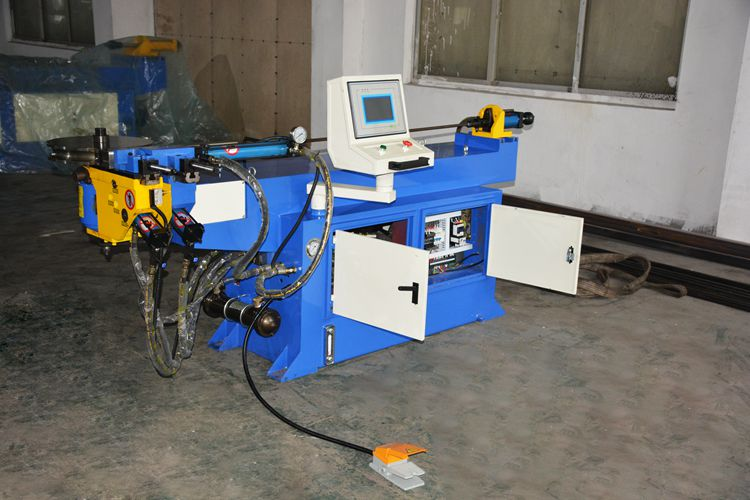According to the knowledge in the "elastoplastic mechanics" textbook.

This is a moment balance equation with the external force moment on the left and the internal force moment on the right, which is equal to the integral of the entire section of the moment generated by the stress on the neutral axis. When bent, the axis of the tube is the central axis.

If the outer diameter D, the inner diameter d, and the yield limit σs of the round pipe are deformed by the bent pipe on the master having the radius of curvature R, the inner ring radius of the pipe is R. The distance between the point where the tube is bent and deformed is L, and the material is not hardened. When the tube is deformed, the entire clean surface enters a plastic state.

Therefore: P*L=2*Integral{2*x*dy*σs*y,0,D/2}-2*Integral{2*x*dy*σs*y,0,d/2}

={D^3-d^3}*σs/6

P={D^3-d^3}*σs/[6*L]

This is the amount of bending force when the material is not hardened.